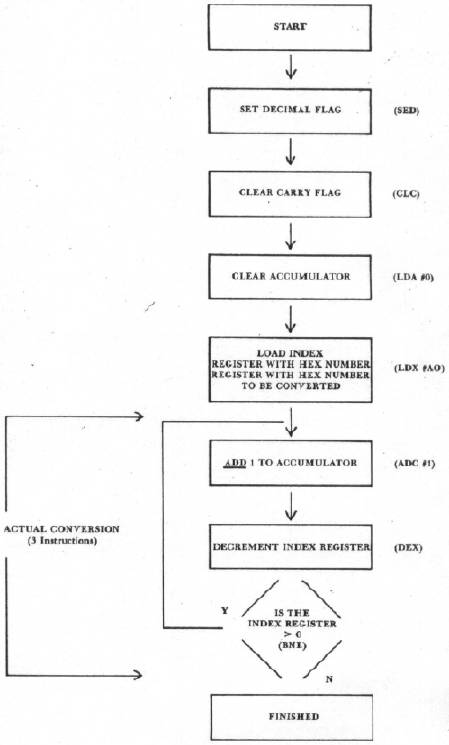COMPUTE! ISSUE 10 / MARCH 1981 / PAGE 60

 Hex Conversion Using The 6502's Decimal Mode Jack Clarke Since the advent of 8 bit microprocessors, the hexidecimal numbering system has been around to help provide a shorthand notation for binary numbers... remember 4 binary bits can be expressed with just 1 hexadecimal character? (F = 11112) While this shorthand notation has revolutionized Assembly Language coding, undoubtedly many a new computerist has cursed the notation as problematical, confusing and cumbersome. To assist the programmer (old and new), elaborate tables have been generated to convert the radix of a number from one base to another... remember radix and base are synonymous? To further the cause of this translation, numerous programs have been written in higher level languages. Take a look at Texas Instrument's hand-held "Programmer" which has gained a commendable respect in the programming community. Have you ever tried to poke or peek with your Apple without one of the above? What is this decimal mode you ask? Simply defined it is a clever bit of binary manipulation that is performed inside the microprocessor to insure that when you add, a "1" to a "9" that the result is "θ with carry" and not "A", (also known as BCD coding). In other words, 4 binary bits can express a decimal number 0 thru 9, (10 thru 15 is illegal). So an eight bit number provides numbering 0 thru 99. Now, let's take a closer look at the 6502's instruction set and see how the decimal mode can help with this numbering conversion. A "bit" of examination reveals that the decimal mode only works when performing an add (ADC) or subtract (SBC) instruction. All other instructions simply ignore the decimal mode. Take for example the increment/decrement instruction. It performs an addition or subtraction (by one) but always in the binary/hex mode. Now, what would happen if we combined a decrement/increment instruction with an add/subtract instruction. The increment instruction would count up one in hexadecimal while the add instruction would simultaneously count up in decimal... did I just see a hex to decimal conversion go by? How about an example? Suppose you wish to convert the hex number "A0" to the equivalent decimal number. (Don't pull out your conversion tables yet). Follow the flow chart in Figure 1 and walk through the steps. First set the decimal mode (SED), clear the accumulator (LDA θ IMM) and clear the carry flag (CLC). Next, load the x-register with the hex number to be converted (LDX A0 IMM). Now, the conversion starts. Decrement the x-register (DEX) and test for zero (BNE). If the x-register is >0 then add 1 to the accumulator (ADC 1 IMM). Repeat the sequence until the x-register has counted down to 0. When you examine the contents of the accumulator you will find the decimal equivalent of "A0" sitting there quietly. If you need a hex equivalent of a decimal number you would enter the decimal number in the accumulator and subtract one in the decimal mode... each time you subtract you would also increment the x-register. See any similarities? For numbers greater than 99 you would perform the addition or subtraction using two or more memory locations and keep track of the carry flag, (double precision arithmetic). The X and Y registers could also be cascaded for extended range with 16 bits. Conversion of 0000 thru FFFF could be easily implemented.Hex To Decimal Conversion The best way to familiarize yourself with this type of approach is to try it on your own computer. After gaining a little confidence in the ease of the conversion, you will soon find the same techniques incredibly helpful in more complex operations such as multiplication and division. Take the example of a program that is sampling the rate of an asynchronous input... By knowing the "sample time" of your program (each time you read the port) and adding that constant instead of "1" you effectively convert and multiply in one operation resulting in a decimal formatted "total number of samples" To summarize the concept of radix conversion using the 6502's decimal mode, start with zero in the accumulator and index register and add "1" to the accumulator (decimal mode) and increment the index register at the same time. You will observe the accumulator counting up in decimal and the index register counting up in binary/hex. Say good-bye to those dog-eared tables and long involved conversion programs that you have been using. The 6502 takes another bow.